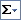# Summary functions available in a PivotTable list

The following table lists the summary functions provided by the AutoCalccommand that you use to create total fields.

Function Description
Sum Calculates the sum of the values.
Count Tallies the number of values. Empty values, such as zero-length strings (strings that contain no characters), are included in the count, but null values (values that indicate missing or unknown data) are not included.
Min Returns the smallest value in the field being summarized.
Max Returns the largest value in the field being summarized.
Average Returns the average of the values in the field being summarized.
Standard Deviation Returns the standard deviation of the values in the field being summarized, assuming the values are a sample of a larger population.
Standard Deviation Population Returns the standard deviation of the values in the field being summarized, assuming the values are the entire population.
Variance Returns the variance of the values in the field being summarized, assuming the values are a sample of a larger population.
Variance Population Returns the variance of the values in the field being summarized, assuming the values are the entire population.

Note  The summary functions available for a field depend on the type of data in the field. For example, if a field contains text, you cannot use Sum, Min, or Max, but you can use Count for that field.